Total marks: --
Total time: --
INSTRUCTIONS
(1) Assume appropriate data and state your reasons
(2) Marks are given to the right of every question
(3) Draw neat diagrams wherever necessary

Answer any one question from Q1 and Q2
1 (a) Obtain Thevenin and Norton equivalent circuits for the network shown in fig. 1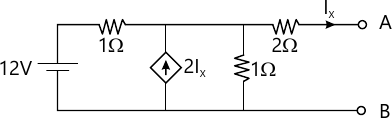6 M
1 (b) For the graph and tree given in Fig. 2. find complete incidence matrix, tieset matrix and F-cutset matrix: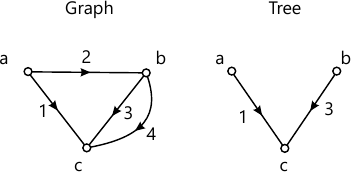6 M

2 (a) For the network shown in Fig. 3, determine the current I2 using superposition theorem.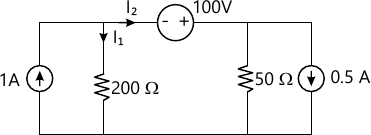6 M
2 (b) For the given incidence matrix, draw oriented graph and determine number of possible trees. $A=\begin{bmatrix} -1&\ 1 &\ 1 &\ 0 &\ 0 &\ 0 \\ \ 0 &-1 &\ 0&-1 &\ 1 & \ 0 \\ \ 0 &\ 0 &-1 &\ 1 &\ 0 &\ 1 \end{bmatrix}$
6 M

Answer any one question from Q3 and Q4
3 (a) Find the expression for Vc(t) in the network shown in Fig .4.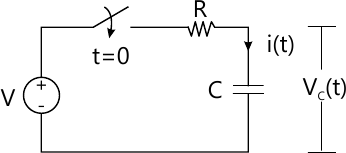6 M
3 (b) A series resonant circuit consists of R=10Ω, L=100 mH and C=10 nF. Find resonant frequency ωr, Fr, quality factor Qr at resonant frequency, bandwidth. Also find current flowing through circuit at resonance if the applied voltage is 100V.
6 M

4 (a) For the network shown in Fig. 5, obtain the expression for iL(t).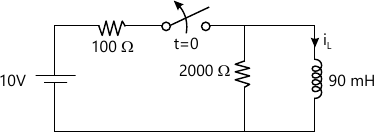6 M
4 (b) A parallel resonant circuit has a coil of 100 μH with Q factor of 100 and is resonated at 1 MHz. Find:
i) Capacitance
ii)o Resistance of coil
iii) Bandwidth
iv) Impedance at parallel resonance.
6 M

Answer any one question from Q5 and Q6
5 (a) A Pi-section constant K filter consists of series arm inductance of 20 mH and two shunt arm capacitors of 0.1 μF each. Calculate cut-off frequency, attenuation at 1.5 kHz. Also find nominal impedance Zπ at f=0 and f=fc.
7 M
5 (b) For a T-section symmetrical network derive the expression for Zoc, Zsc and characteristic impedance Zo.
6 M

6 (a) For the system with 500 Ω resistance design T and Pi attenuators to have 100 dB attenuation. Also draw T and Pi attenuators showing the designed component values.
7 M
6 (b) Draw the T section and Pi section contant K Band pass filter circuits and write equations for components in series arm and shunt arm
6 M

Answer any one question from Q7 and Q8
7 (a) Explain different network functions for one port and two port networks.
7 M
7 (b) Determine Z parameters for the network shown in Fig 6.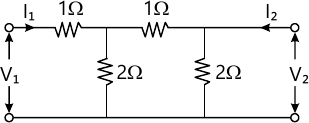6 M

8 (a) Determine the impedance function Z(s) for the network shown Fig. 7. Also draw its pole zero plot.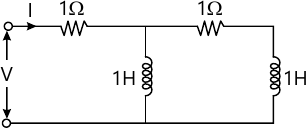7 M
8 (b) Determine Y parameters of the network shown in Fig. 8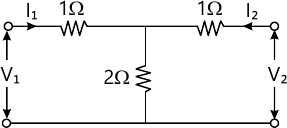6 M

More question papers from Network Theory